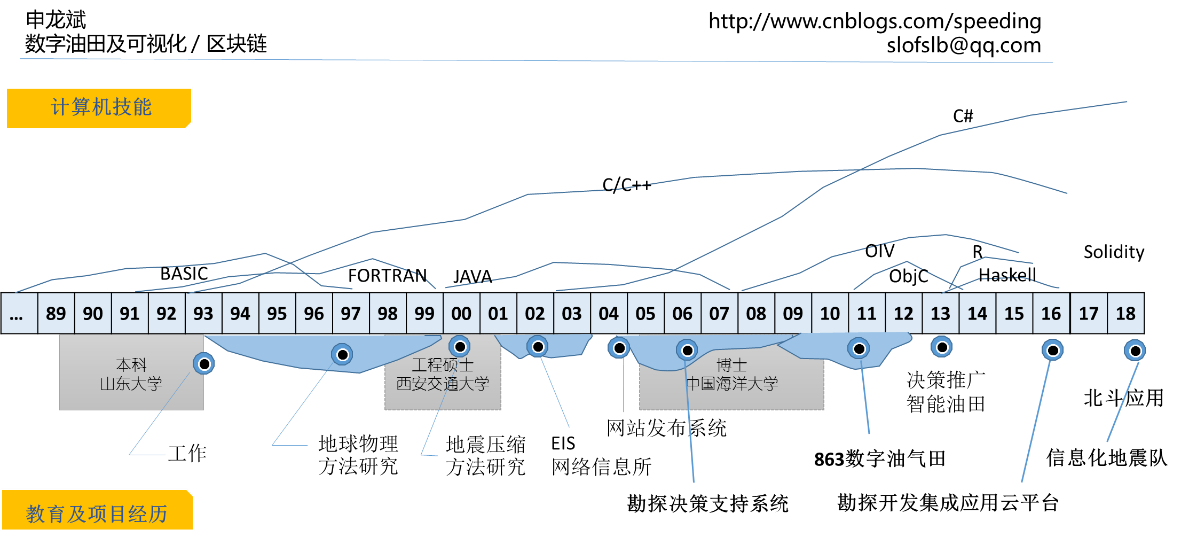# 通过欧拉计划学习Rust编程（第22~25题）

## 第22题

1）读文件，移除引号

2）把名字存储在Vec向量中

3）排序

4）求字符在字母表中的序号

5）求单词的分数

6）求总分

1）首先把文件读到一个字符串中。

use std::fs;

fn main() {
.expect("读文件失败");
println!("{}", data);
}


fn remove_quote(s: &str) -> String {
s.chars().filter(|c| *c !='"').collect()
}


2）每个名字是用逗号分开的，所以可以用split()函数，分解成向量。

let data2 = remove_quote(&data);
let names: Vec<&str> = data2.split(",").collect();
println!("{:?}", names);


3）向量有专门的排序函数，需要将变量定义为可修改的。

let mut names: Vec<&str> = data2.split(",").collect();
names.sort();


4）字符在字母表中的顺序号，可以求find()，也可以用position()函数。

fn letter_number(ch: char) -> usize {
let letters = "ABCDEFGHIJKLMNOPQRSTUVWXYZ";
letters.chars().position(|c| c == ch).unwrap() + 1
}


5）求一个单词的分数

fn word_score(word: &str) -> usize {
let mut score = 0;
for ch in word.chars() {
score += letter_number(ch);
}
score
}


6）现在可以求总分了，有一个非常有用的for循环的用法，可以既得到元素，还可以得到元素的索引号，利用enumerate()函数。

let mut score = 0;
for (i, name) in names.iter().enumerate() {
let ws = word_score(name);
println!("{} {} {}", (i+1), name, ws);
score += ws * (i + 1);
}
println!("{}", score);


let data = std::fs::read_to_string("names.txt").expect("读文件失败");
let data2 = remove_quote(&data);
let mut names: Vec<&str> = data2.split(",").collect();

names.sort();

let mut score = 0;
for (i, name) in names.iter().enumerate() {
let ws = word_score(name);
println!("{} {} {}", (i + 1), name, ws);
score += ws * (i + 1);
}
println!("{}", score);


1）std::fs读文件

2）字符串的split()函数

3）排序函数sort()

4）字符串中查找一个字符的位置

5）enumerate()迭代器，可以产生序号和元素

## 第23题

1）求所有因子（不包含自身）

2）判断是否为富裕数

3）判断是否可以分解为2个富裕数之和

4）求解最后的问题

fn proper_divisors(num: u32) -> Vec<u32> {
let mut v = { // 求一半的因子
let s = (num as f32).sqrt() as u32;
(1..=s).filter(|x| num % x == 0).collect::<Vec<u32>>()
};
let last = v.last().unwrap();
if last * last == num {
// 16的一半因子为1,2,4，另外只差一个8，即16 / 2
for i in (1..v.len()-1).rev() {
v.push(num / v[i]);
}
}
else {
// 12的一半因子为1,2,3，另外一半因子：4,6，分别对应于12/3，12/2
for i in (1..v.len()).rev() {//不要num自身，所以从1开始
v.push(num / v[i]);
}
}
v
}


fn is_abundant_number(num: u32) -> bool {
let proper_divisors_sum = proper_divisors(num).iter().sum::<u32>();
proper_divisors_sum > num
}


let mut abundant_numbers = vec![false; 28124];
for i in 2usize..abundant_numbers.len() {
if is_abundant_number(i as u32) {
abundant_numbers[i] = true;
}
}


fn can_divide(abundant_numbers: &[bool], num: u32) -> bool {
for x in 1..=28123 {
let y = num - x;
if y <= 0 {break;}
if abundant_numbers[x as usize] && abundant_numbers[y as usize] {
// println!("{} = {} + {}", num, x, y);
return true;
}
}
return false;
}


let mut sum = 0;
for i in 1..=28123 {
if !can_divide(&abundant_numbers, i) {
sum += i;
}
}
println!("sum: {}", sum);


println!("sum: {}",
(1..=28123).filter(|&x| !can_divide(&abundant_numbers, x))
.sum::<u32>()
);


• 数组作为函数参数的写法：&[bool]

## 第24题

0，1，2，3，4，5，6，7，8和9，每个数字用且只用一次，称为全排列，按数值大小排序，求第一百万个数是多少？fn next_perm(v: &mut Vec<u32>) {
let mut i = v.len() - 2;
while v[i] > v[i + 1] {
i -= 1;
}

let mut j = v.len() - 1;
while i < j && v[i] > v[j] {
j -= 1;
}

swap(v, i, j);

i += 1;
j = v.len() - 1;
while i < j {
swap(v, i, j);
i += 1;
j -= 1;
}
}

fn swap(v: &mut Vec<u32>, i: usize, j: usize) {
let temp = v[i];
v[i] = v[j];
v[j] = temp;
}



fn main() {
let mut v: Vec<u32> = vec![0, 1, 2, 3, 4, 5, 6, 7, 8, 9];
for i in 2..=1_000_000 {
next_perm(&mut v);
//println!("{} {:?}", i, v);
}
println!("{:?}", v);
}


let v_str = v.iter()
.map(|x| x.to_string())
.collect::<String>();
println!("{}", v_str.parse::<u64>().unwrap());


fn main() {
let mut v = [0, 1, 2, 3, 4, 5, 6, 7, 8, 9];
for i in 2..=1_000_000 {
next_perm(&mut v);
}
println!("{:?}", v);
}

fn next_perm(v: &mut [u32]) {
// 保持不变
}

fn swap(v: &mut [u32], i: usize, j: usize) {
let temp = v[i];
v[i] = v[j];
v[j] = temp;
}


• 注意向量或数组传递到函数里的写法

## 第25题

extern crate num_bigint;
use num_bigint::BigUint;

fn main() {
let mut prev = BigUint::from(1 as u64);
let mut cur = BigUint::from(1 as u64);
for i in 3.. {
let next = prev + &cur;
let str = next.to_string();
if str.len() >= 1000 {
println!("{} {} {}", i, str, str.len());
break;
}
prev = cur;
cur = next;
}
}


1539870_KBNiIXymh4SnmDEDZmUTg7tu1MTBVlLj

----==== Email: slofslb (GTD) qq.com 请将(GTD)换成@ ====----

---- 魔方桥牌象棋、游戏人生...